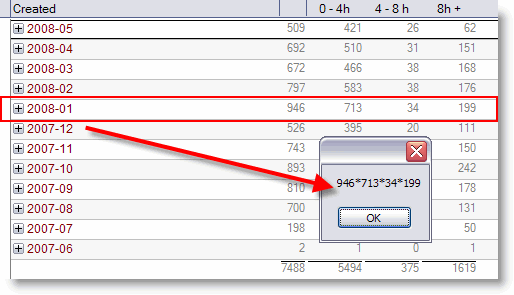# Get View Column Sums With LotusScript

I surfed the web the other day and came across an entry in OpenNTF’s codebin.  Ferry Kranenburg posted a sample database that shows, how you can create graphs using RMchart from Notes Views.

In his code,  Ferry uses the NotesViewNavigator class to search a view for a given key and to return the according view column values as a string when the search returns a match.

The original function (= var_FastViewLookup ) is very static, because the columns to return are hardcoded, so it cannot be used in other applications without modifications. So I decided to rewrite the function to be more generic. Here is the result:

```Function var_FastViewLookup(_
db As NotesDatabase,_
View As String,_
Key As String,_
KeyCol As Integer,_
cols As Variant) As Variant

On Error Goto Err_Handle
Dim nav As NotesViewNavigator
Dim ve As NotesViewEntry
Dim v As NotesView
Dim i As Integer
Redim colVal (1) As String
If Trim(view) = "" Then Goto Exit_Here

Redim colVal (Ubound(cols)) As String
Set v = db.GetView(view)

Set nav = v.CreateViewNav
Set ve = nav.GetFirst

While Not ve Is Nothing
If ve.ColumnValues(KeyCol) = Key Then
i = 0
Forall c In cols
colVal(i) = ve.ColumnValues(c)
i = i+1
End Forall
Set ve = Nothing
Else
Set ve = nav.GetNextCategory(ve)
End If
Wend
Exit_Here:
var_FastViewLookup = colVal
Exit Function
Err_Handle:
Resume Exit_Here
End Function```

Here is a sample of how to use the function:

```Sub Click(Source As Button)
Dim s As New NotesSession
Dim db As NotesDatabase
Set db = s.CurrentDatabase
Dim retval As String

Dim KeyCol  As Integer
KeyCol = 2 ' View column containing category search key

Dim cols (3) As Integer
cols(0) = 7 ' all tickets ( sum )
cols(1) = 8 ' 0 - 4h
cols(2) = 9 ' 4 - 8h
cols(3) = 10 ' 8h +

retval = Join(_
var_FastViewLookup(db , "(\$ddTickets_ByMonthRep)", "2008-01", KeyCol, cols), "*")

Msgbox retval
End Sub```

The sample returns the following value## One thought on “Get View Column Sums With LotusScript”

1.Ferry Kranenburg

Thanks you for the rewritten function. I updated my example at openntf.

I was thinking, we could try to rewrite the function and change the keycolumn parameter into an array just like you did with the cols parameter. Sometimes views do have 5 subcategories and 1 totals column. What if you would like the totals of the 3rd subcategory? It will save some extra views right?